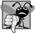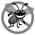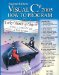# Logical Operators

The if, if...else, while, do...while and for statements each require a condition to determine how to continue an application's flow of control. So far, we have studied only simple conditions, such as count <= 10, number != sentinelValue and total > 1000. Simple conditions are expressed in terms of the relational operators >, <, >= and <=, and the equality operators == and !=. Each expression tests only one condition. To test multiple conditions in the process of making a decision, we performed these tests in separate statements or in nested if or if...else statements. Sometimes, control statements require more complex conditions to determine an application's flow of control.

C# provides logical operators to enable you to form more complex conditions by combining simple conditions. The logical operators are && (conditional AND), || (conditional OR), & (boolean logical AND), | (boolean logical inclusive OR), ^ (boolean logical exclusive OR) and ! (logical negation).

Conditional AND (&&) Operator

Suppose that we wish to ensure at some point in an application that two conditions are both true before we choose a certain path of execution. In this case, we can use the && (conditional AND) operator, as follows:

```if ( gender == FEMALE && age >= 65 )
seniorFemales++;
```

This if statement contains two simple conditions. The condition gender == FEMALE compares variable gender to constant FEMALE. This might be evaluated, for example, to determine whether a person is female. The condition age >= 65 might be evaluated to determine whether a person is a senior citizen. The if statement considers the combined condition

```gender == FEMALE && age >= 65
```

which is true if and only if both simple conditions are true. If the combined condition is true, the if statement's body increments seniorFemales by 1. If either or both of the simple conditions are false, the application skips the increment. Some programmers find that the preceding combined condition is more readable when redundant parentheses are added, as in:

```( gender == FEMALE ) && ( age >= 65 )
```

The table in Fig. 6.14 summarizes the && operator. The table shows all four possible combinations of false and TRue values for expression1 and expression2. Such tables are called truth tables. C# evaluates all expressions that include relational operators, equality operators or logical operators to bool valueswhich are either true or false.

Figure 6.14. && (conditional AND) operator truth table.

expression1

expression2

expression1 && expression2

false

false

false

false

TRue

false

TRue

false

false

TRue

true

true

Conditional OR (||) Operator

Now suppose that we wish to ensure that either or both of two conditions are true before we choose a certain path of execution. In this case, we use the || (conditional OR) operator, as in the following application segment:

```if ( ( semesterAverage >= 90 ) || ( finalExam >= 90 ) )
Console.WriteLine ( "Student grade is A" );
```

This statement also contains two simple conditions. The condition semesterAverage >= 90 is evaluated to determine whether the student deserves an A in the course because of a solid performance throughout the semester. The condition finalExam >= 90 is evaluated to determine whether the student deserves an A in the course because of an outstanding performance on the final exam. The if statement then considers the combined condition

```( semesterAverage >= 90 ) || ( finalExam >= 90 )
```

and awards the student an A if either or both of the simple conditions are true. The only time the message "Student grade is A" is not printed is when both of the simple conditions are false. Figure 6.15 is a truth table for operator conditional OR (||). Operator && has a higher precedence than operator ||. Both operators associate from left to right.

Figure 6.15. || (conditional OR) operator truth table.

expression1

expression2

expression1 || expression2

false

false

false

false

TRue

true

true

false

TRue

TRue

TRue

true

Short-Circuit Evaluation of Complex Conditions

The parts of an expression containing && or || operators are evaluated only until it is known whether the condition is true or false. Thus, evaluation of the expression

```( gender == FEMALE ) && ( age >= 65 )
```

stops immediately if gender is not equal to FEMALE (i.e., at that point, it is certain that the entire expression is false) and continues if gender is equal to FEMALE (i.e., the entire expression could still be true if the condition age >= 65 is true). This feature of conditional AND and conditional OR expressions is called short-circuit evaluation.## Common Programming Error 6 8

In expressions using operator &&, a conditionwhich we refer to as the dependent conditionmay require another condition to be true for the evaluation of the dependent condition to be meaningful. In this case, the dependent condition should be placed after the other condition, or an error might occur. For example, in the expression ( i != 0 ) && ( 10/ i == 2 ), the second condition must appear after the first condition, or a divide-by-zero error might occur.

Boolean Logical AND (&) and Boolean Logical OR (|) Operators

The boolean logical AND (&) and boolean logical inclusive OR (|) operators work identically to the && (conditional AND) and || (conditional OR) operators, with one exceptionthe boolean logical operators always evaluate both of their operands (i.e., they do not perform short-circuit evaluation). Therefore, the expression

```( gender == 1 ) & ( age >= 65 )
```

evaluates age >= 65 regardless of whether gender is equal to 1. This is useful if the right operand of the boolean logical AND or boolean logical inclusive OR operator has a required side effecta modification of a variable's value. For example, the expression

```( birthday == true ) | ( ++age >= 65 )
```

guarantees that the condition ++age >= 65 will be evaluated. Thus, the variable age is incremented in the preceding expression, regardless of whether the overall expression is TRue or false.## Error Prevention Tip 6 5

For clarity, avoid expressions with side effects in conditions. The side effects may look clever, but they can make it harder to understand code and can lead to subtle logic errors.

Boolean Logical Exclusive OR (^)

A complex condition containing the boolean logical exclusive OR (^) operator (also called the logical XOR operator) is true if and only if one of its operands is TRue and the other is false. If both operands are true or both are false, the entire condition is false. Figure 6.16 is a truth table for the boolean logical exclusive OR operator (^). This operator is also guaranteed to evaluate both of its operands.

Figure 6.16. ^ (boolean logical exclusive OR) operator truth table.

expression1

expression2

expression1 ^ expression2

false

false

false

false

TRue

TRue

TRue

false

true

true

TRue

false

Logical Negation (!) Operator

The ! (logical negation) operator enables you to "reverse" the meaning of a condition. Unlike the logical operators &&, ||, &, | and ^, which are binary operators that combine two conditions, the logical negation operator is a unary operator that has only a single condition as an operand. The logical negation operator is placed before a condition to choose a path of execution if the original condition (without the logical negation operator) is false, as in the code segment

```if ( ! ( grade == sentinelValue ) )
```

which executes the WriteLine call only if grade is not equal to sentinelValue. The parentheses around the condition grade == sentinelValue are needed because the logical negation operator has a higher precedence than the equality operator.

In most cases, you can avoid using logical negation by expressing the condition differently with an appropriate relational or equality operator. For example, the previous statement may also be written as follows:

```if ( grade != sentinelValue )
```

This flexibility can help you express a condition in a more convenient manner. Figure 6.17 is a truth table for the logical negation operator.

Figure 6.17. ! (logical negation) operator truth table.

expression

!expression

false

TRue

TRue

false

Logical Operators Example

Figure 6.18 demonstrates the logical operators and boolean logical operators by producing their truth tables. The output shows the expression that was evaluated and the bool result of that expression. Lines 1014 produce the truth table for && (conditional AND). Lines 1721 produce the truth table for || (conditional OR). Lines 2428 produce the truth table for & (boolean logical AND). Lines 3136 produce the truth table for | (boolean logical inclusive OR). Lines 3944 produce the truth table for ^ (boolean logical exclusive OR). Lines 4749 produce the truth table for ! (logical negation).

Figure 6.18. Logical operators.

(This item is displayed on pages 251 - 252 in the print version)

``` 1 // Fig. 6.18: LogicalOperators.cs
2 // Logical operators.
3 using System;
4
5 public class LogicalOperators
6 {
7 public static void Main( string[] args )
8 {
9 // create truth table for && (conditional AND) operator
10 Console.WriteLine( "{0}
{1}: {2}
{3}: {4}
{5}: {6}
{7}: {8}
",
11 "Conditional AND (&&)", "false && false", ( false && false ),
12 "false && true", ( false && true )
13 "true && false", ( true && false ),
14 "true && true", ( true && true ) );
15
16 // create truth table for || (conditional OR) operator
17 Console.WriteLine( "{0}
{1}: {2}
{3}: {4}
{5}: {6}
{7}: {8}
",
18 "Conditional OR (||)", "false || false", ( false || false ),
19 "false || true", ( false || true ),
20 "true || false", ( true || false ),
21 "true || true", ( true || true ) );
22
23 // create truth table for & (boolean logical AND) operator
24 Console.WriteLine( "{0}
{1}: {2}
{3}: {4}
{5}: {6}
{7}: {8}
",
25 "Boolean logical AND (&)", "false & false", ( false & false )
26 "false & true", ( false & true ),
27 "true & false", ( true & false ),
28 "true & true", ( true & true ) );
29
30 // create truth table for | (boolean logical inclusive OR) operator
31 Console.WriteLine( "{0}
{1}: {2}
{3}: {4}
{5}: {6}
{7}: {8}
",
32 "Boolean logical inclusive OR (|)",
33 "false | false", ( false | false ),
34 "false | true", ( false | true ),
35 "true | false", ( true | false ),
36 "true | true", ( true | true ) );
37
38 // create truth table for ^ (boolean logical exclusive OR) operator
39 Console.WriteLine( "{0}
{1}: {2}
{3}: {4}
{5}: {6}
{7}: {8}
",
40 "Boolean logical exclusive OR (^)",
41 "false ^ false", ( false ^ false ),
42 "false ^ true", ( false ^ true ),
43 "true ^ false", ( true ^ false ),
44 "true ^ true", ( true ^ true ) );
45
46 // create truth table for ! (logical negation) operator
47 Console.WriteLine( "{0}
{1}: {2}
{3}: {4}",
48 "Logical negation (!)", "!false", ( !false ),
49 "!true", ( !true ) );
50 } // end Main
51 } // end class LogicalOperators
```

 ```Conditional AND (&&) false && false: False false && true: False true && false: False true && true: True Conditional OR (||) false || false: False false || true: True true || false: True true || true: True Boolean logical AND (&) false & false: False false & true: False true & false: False true & true: True Boolean logical inclusive OR (|) false | false: False false | true: True true | false: True true | true: True Boolean logical exclusive OR (^) false ^ false: False false ^ true: True true ^ false: True true ^ true: False Logical negation (!) !false: True !true: False ```

Figure 6.19 shows the precedence and associativity of the C# operators introduced so far. The operators are shown from top to bottom in decreasing order of precedence.

Figure 6.19. Precedence/associativity of the operators discussed so far.

(This item is displayed on pages 252 - 253 in the print version)

Operators

Associativity

Type

```. new ++(postfix) --(postfix)
```

left to right

highest precedence

```++ -- + - ! (type)
```

right to left

unary prefix

```* / %
```

left to right

multiplicative

`+ -`

left to right

```< <= > >=
```

left to right

relational

```== !=
```

left to right

equality

&

left to right

boolean logical AND

^

left to right

boolean logical exclusive OR

|

left to right

boolean logical inclusive OR

&&

left to right

conditional AND

||

left to right

conditional OR

?:

right to left

conditional

```= += -= *= /= %=
```

right to left

assignmentVisual C# 2005 How to Program (2nd Edition)
ISBN: 0131525239
EAN: 2147483647
Year: 2004
Pages: 600